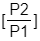# Problem: A sample of a gas (1.50 mol) is contained in a 15 0 L cylinder The temperature is increased from 100 °C to 150 °C.The ratio of final pressure to initial pressure  is ___.A) 1.50 B) 0.667 C) 1.13 D) 1.00 E) 0.882

###### FREE Expert Solution

Pressure is directly proportional to temperature:

$\overline{)\frac{{\mathbf{P}}_{\mathbf{1}}}{{\mathbf{T}}_{\mathbf{1}}}{\mathbf{=}}\frac{{\mathbf{P}}_{\mathbf{2}}}{{\mathbf{T}}_{\mathbf{2}}}}\phantom{\rule{0ex}{0ex}}\frac{{\mathbf{P}}_{\mathbf{1}}}{{\mathbf{P}}_{\mathbf{2}}}\mathbf{=}\frac{{\mathbf{T}}_{\mathbf{1}}}{{\mathbf{T}}_{\mathbf{2}}}$

90% (71 ratings)###### Problem Details

A sample of a gas (1.50 mol) is contained in a 15 0 L cylinder The temperature is increased from 100 °C to 150 °C.The ratio of final pressure to initial pressureis ___.

A) 1.50

B) 0.667

C) 1.13

D) 1.00

E) 0.882